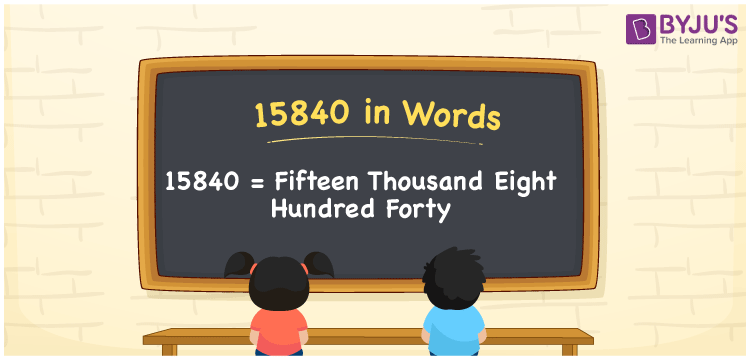# 15840 in words

15840 in words is written as Fifteen Thousand Eight Hundred and Forty. In 15840, 1 has a place value of ten thousand, 5 is in the place value of thousand, 8 is in the place value of hundred and 4 is in the place value of ten. The article on Place Value gives more information. The number 15840 is used in expressions that relate to money, distance, social media views, and many more. For example, “There are Fifteen Thousand Eight Hundred and Forty people in the guest list for a wedding reception.”

 15840 in words Fifteen Thousand Eight Hundred and Forty Fifteen Thousand Eight Hundred and Forty in Numbers 15840

## 15840 in English Words## How to Write 15840 in Words?

We can convert 15840 to words using a place value chart. The number 15840 has 5 digits, so let’s make a chart that shows the place value up to 5 digits.

 Ten thousand Thousands Hundreds Tens Ones 1 5 8 4 0

Thus, we can write the expanded form as:

1 × Ten thousand + 5 × Thousand + 8 × Hundred + 4 × Ten + 0 × One

= 1 × 10000 + 5 × 1000 + 8 × 100 + 4 × 10 + 0 × 1

= 15840.

= Fifteen Thousand Eight Hundred and Forty.

15840 is the natural number that is succeeded by 15839 and preceded by 15841.

15840 in words – Fifteen Thousand Eight Hundred and Forty.

Is 15840 an odd number? – No.

Is 15840 an even number? – Yes.

Is 15840 a perfect square number? – No.

Is 15840 a perfect cube number? – No.

Is 15840 a prime number? – No.

Is 15840 a composite number? – Yes.

## Solved Example

1. Write the number 15840 in expanded form

Solution: 1 x 10000 + 5 x 1000 + 8 x 100 + 4 x 10 + 0 x 1

Or Just 1 x 10000 + 5 x 1000 + 8 x 100 + 4 x 10

We can write 15840 = 10000 + 5000 + 800 + 40 + 0

= 1 x 10000 + 5 x 1000 + 8 x 100 + 4 x 10 + 0 x 1.

## Frequently Asked Questions on 15840 in words

Q1

### How to write the number 15840 in words?

15840 in words is written as Fifteen Thousand Eight Hundred and Forty.
Q2

### State whether True or False. 15840 is divisible by 3?

True. 15840 is divisible by 3.
Q3

### Is 15840 divisible by 10?

Yes. 15840 is divisible by 10.